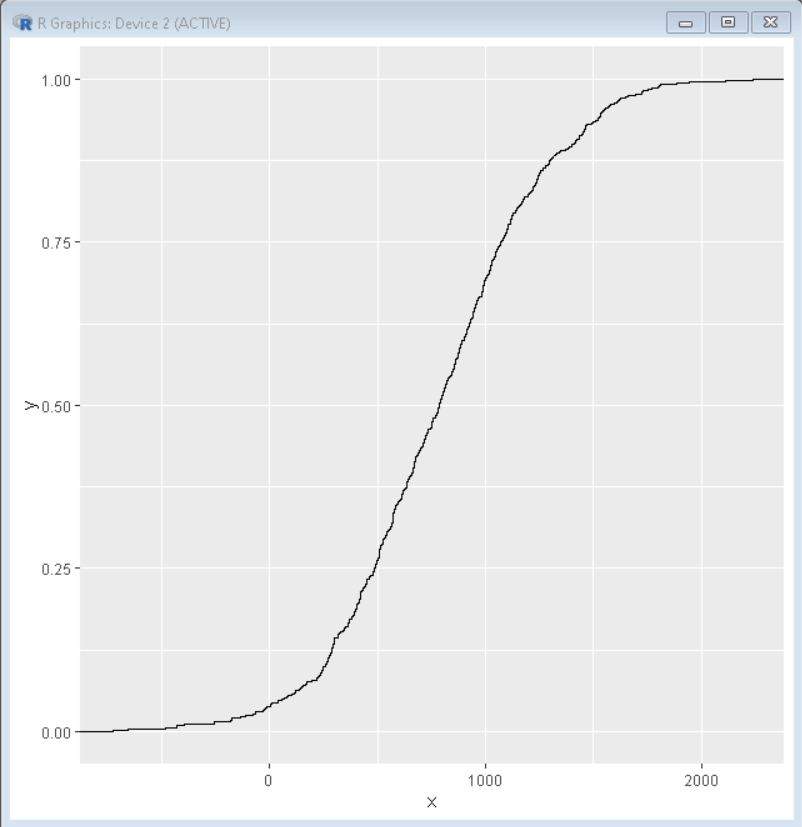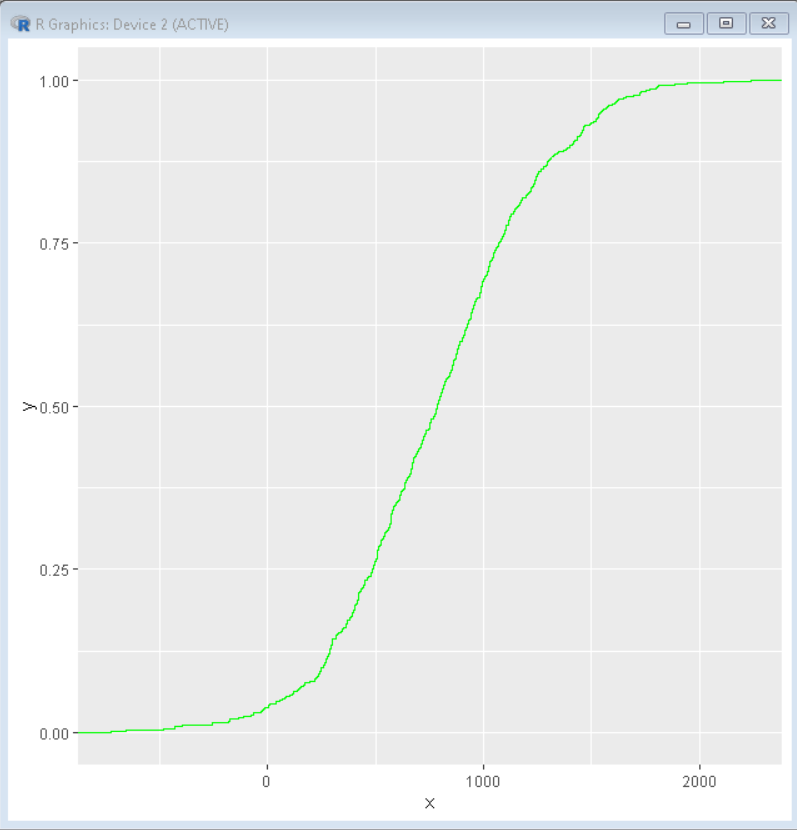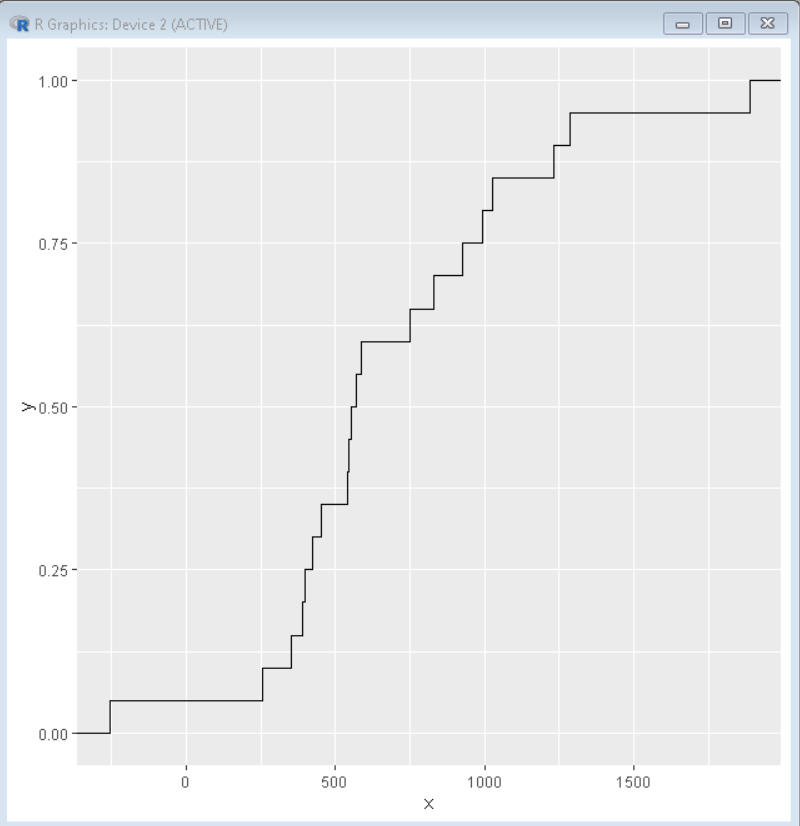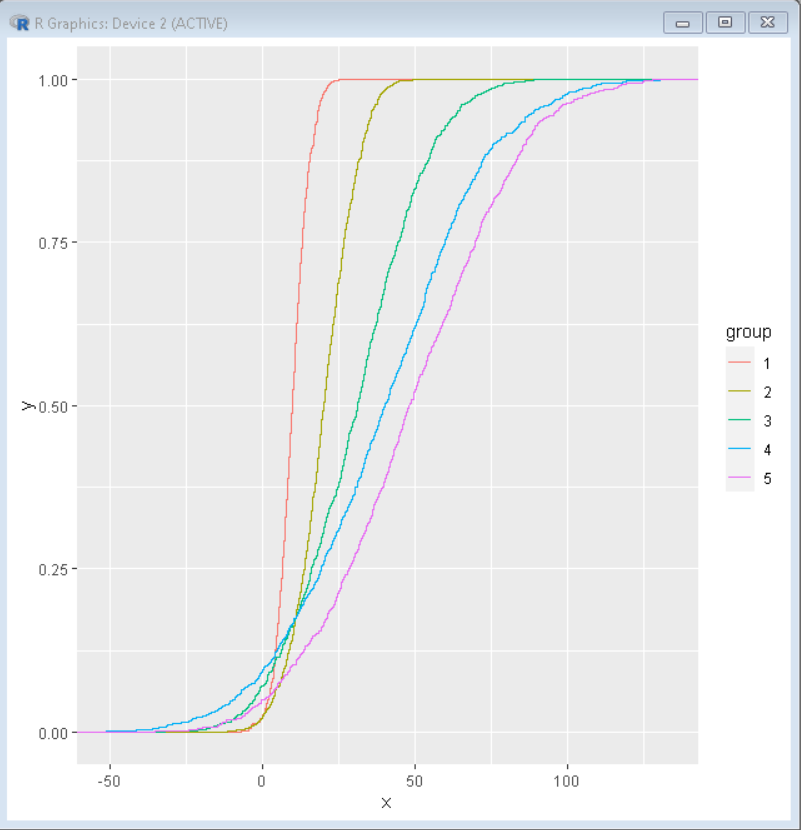# How to Make ECDF Plot with ggplot2 in R?

• Last Updated : 17 Oct, 2021

Empirical Cumulative Distribution Function Plot (ECDF) helps us to visualize one or more distributions. ECDF plot is a great alternative for histograms and it has the ability to show the full range of data without the need for various parameters.

In this article, we will discuss how to draw an ECDF plot using the ggplot2 package of R Programing language. To draw an ECDF plot, we use the stat_ecdf() function of the ggplot2 package of R Language.

Syntax: ggplot( df, aes(x)) + stat_ecdf( geom, col )

Parameters:

• df : determines dataframe used to plot ECDF plot
• geom: determines the shape of plot, i.e., point, step,etc.
• col: determines the color of plot

## Create a basic ECDF plot

In this example, we will create a basic ECDF plot. We will use the ggplot() function and the stat_ecdf() function to plot the ECDF plot.

## R

 `# set seed``set.seed``(1234)`` ` `# create a random data frame ``sample_data <- ``data.frame``(x = ``round``(``rnorm``(700, ``                                          ``mean=800, ``                                          ``sd=450)))`` ` `# load library ggplot2``library``(ggplot2)`` ` `# Basic ECDF plot using ggplot package``ggplot``(sample_data, ``aes``(x)) + `` ` `# stat_ecdf() function is used to plot ECDF plot``stat_ecdf``()`

Output:## Color Customization

To change the color of the ECDF plot we use the col parameter of the stat_ecdf() function. We can add any color as the value of parameter col. We can even use hex codes of color. In this example, we have a green-colored ECDF plot made using stat_ecdf() function with the col parameter being green.

## R

 `# set seed``set.seed``(1234)`` ` `# create a random data frame ``sample_data <- ``data.frame``(x = ``round``(``rnorm``(700, mean=800, ``                                          ``sd=450)))`` ` `# load library ggplot2``library``(ggplot2)`` ` `# Basic ECDF plot using ggplot package``ggplot``(sample_data, ``aes``(x)) + `` ` `# stat_ecdf() function is used to plot ECDF plot``# col parameter is used to color plot as green``stat_ecdf``(col=``"green"``)`

Output:## Shape Customization

To change the shape of the ECDF plot we use the geom parameter of the stat_ecdf() function. We can add any shape as the value of parameter geom. In this example, we have a stair-shaped ECDF plot made using stat_ecdf() function with the geom parameter being “step”.

## R

 `# set seed``set.seed``(1234)`` ` `# create a random data frame ``sample_data <- ``data.frame``(x = ``round``(``rnorm``(20, mean=800, sd=450)))`` ` `# load library ggplot2``library``(ggplot2)`` ` `# Basic ECDF plot using ggplot package``ggplot``(sample_data, ``aes``(x)) + `` ` `# stat_ecdf() function is used to plot ECDF plot``# geom parameter is used to shape plot as step``stat_ecdf``(geom=``"step"``)`

Output:## Multiple ECDF colored by group:

ECDF plot can be used for plotting multiple distributions. To plot multiple ECDF plots, we firstly create a multi-dimension dataset and then use the col parameter of the aes() function to color them according to the group.

Here, is a multiple distribution ECDF plots plotted with color by group using col parameter of the aes() function of the ggplot2 package. To create a multi-dimension dataset we will use rnorm() functions with gl() function to group them in 5 columns of size 1000.

## R

 `# set seed``set.seed``(1234)`` ` `# create a random data frame ``sample_data <- ``data.frame``(x = ``c``(``rnorm``(1000, 10, 5), ``                        ``rnorm``(1000, 20, 10),``                        ``rnorm``(1000, 30, 20),``                        ``rnorm``(1000, 40, 30),``                        ``rnorm``(1000, 50, 30)),``                          ``group = ``gl``(5, 1000))`` ` `# load library ggplot2``library``(ggplot2)`` ` `# Basic ECDF plot using ggplot package``# col parameter is used to color plot ``# according to group``ggplot``(sample_data, ``aes``(x=x, col=group)) + `` ` `# stat_ecdf() function is used to plot ECDF plot``stat_ecdf``()`

Output:My Personal Notes arrow_drop_up